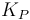# Difference between revisions of "Theory of Operation"

## Control Loops Writeup

Excellent explanation of the Control Loops and some basics on Control Theory behind it.

## PID

Paparazzi uses common Proportional Integral Derivative (PID) control for stability and navigation. PID is probably the most commonly used feedback control design as it is simple to implement and intuitive to operate. PID controllers use three terms operating on the measured error to produce a control output. If u(t) is the control signal sent to the system, y(t) is the measured output and r(t) is the desired output, and tracking error$e(t)=r(t)- y(t)$, a PID controller has the general form$u(t) = K_P e(t) + K_I \int e(t)dt + K_D \dot{e}(t)$

The desired closed loop dynamics are obtained by adjusting the three parameters$K_P$,$K_I$ and$K_D$, often iteratively by "tuning" and without specific knowledge of a plant model. Stability can often be ensured using only the proportional term in well damped systems. The integral term compensates for steady long-term errors, and the derivative term is used to provide damping to reduce oscillation.

See more on Wikipedia: PID Controller

PID explained by RC Model Reviews: What PIDs do and how they do it

Paparazzi uses PID controllers on all loops but many of the I and D terms are not fully implemented as they are often unnecessary or difficult to tune. Below are some examples of the PID implementations in Paparazzi. There is a graphical description of the control loops as well.

### Roll Rate

 File: In sw/airborne/firmwares/fixedwing/stabilization/stabilization_attitude.c we define the roll rate loop: float cmd = throttle_dep_pgain * ( err + h_ctl_roll_rate_igain * roll_rate_sum_err / H_CTL_ROLL_RATE_SUM_NB_SAMPLES + h_ctl_roll_rate_dgain * d_err); 

Note that the roll Pgain is variable with throttle and multiplies through the entire equation affecting the I and D terms as well for ease of tuning.

### Roll Attitude

 File: In sw/airborne/firmwares/fixedwing/stabilization/stabilization_attitude.c we define the roll attitude gain loop: float err = estimator_phi - h_ctl_roll_setpoint; float cmd = - h_ctl_roll_attitude_gain * err- h_ctl_roll_rate_gain * estimator_p+ v_ctl_throttle_setpoint * h_ctl_aileron_of_throttle; 

### Pitch Angle

 File: In sw/airborne/firmwares/fixedwing/stabilization/stabilization_attitude.c we define the pitch angle loop: float err = estimator_theta - h_ctl_pitch_setpoint; float d_err = err - last_err; last_err = err; float cmd = h_ctl_pitch_pgain * (err + h_ctl_pitch_dgain * d_err) + h_ctl_elevator_of_roll * fabs(estimator_phi); 

Here we use only Proportional as the Derivative is always set to zero. An integral term could prove useful here. Aircraft pitch response is normally very well damped. Those with "plank" style aircraft or other pitch-sensitive designs may benefit from implementing a gyro-based D term.

 File: In sw/airborne/firmwares/fixedwing/stabilization/stabilization_attitude.c we define the navigation loop:  float err = estimator_hspeed_dir - h_ctl_course_setpoint; NormRadAngle(err); float speed_depend_nav = estimator_hspeed_mod/NOMINAL_AIRSPEED; Bound(speed_depend_nav, 0.66, 1.5); float cmd = h_ctl_course_pgain * err * speed_depend_nav; 
 File: In sw/airborne/firmwares/fixedwing/guidance/guidance_v_n.c we compute the desired climb rate: v_ctl_altitude_error = estimator_z - v_ctl_altitude_setpoint; v_ctl_climb_setpoint = v_ctl_altitude_pgain * v_ctl_altitude_error + v_ctl_altitude_pre_climb; 
 File: Then we compute the throttle response: float err = estimator_z_dot - v_ctl_climb_setpoint; float controlled_throttle = v_ctl_auto_throttle_cruise_throttle + v_ctl_auto_throttle_climb_throttle_increment * v_ctl_climb_setpoint + v_ctl_auto_throttle_pgain * (err + v_ctl_auto_throttle_igain * v_ctl_auto_throttle_sum_err);  and pitch response float v_ctl_pitch_of_vz = v_ctl_climb_setpoint * v_ctl_auto_throttle_pitch_of_vz_pgain;